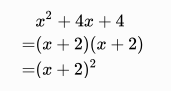# MathJax Newline Alignment Problems in HugoBy Wallpoper [Public domain or Public domain], via Wikimedia Commons

# 一般來說

\begin{aligned}
& x^2+4x+4 \\
=& (x+2)(x+2) \\
=& (x+2)^2
\end{aligned}# 方案？

\begin{aligned}
& x^2+4x+4 \\\\\\
=& (x+2)(x+2) \\\\\\
=& (x+2)^2
\end{aligned}


\begin{aligned} & x^2+4x+4 \\ =&(x+2)(x+2) \\ =&(x+2)^2 \end{aligned}

\begin{aligned}
& x^2+4x+4 \newline
=& (x+2)(x+2) \newline
=& (x+2)^2
\end{aligned}


Render 看看： \begin{aligned} & x^2+4x+4 \newline =&(x+2)(x+2) \newline =&(x+2)^2 \end{aligned} 也好棒